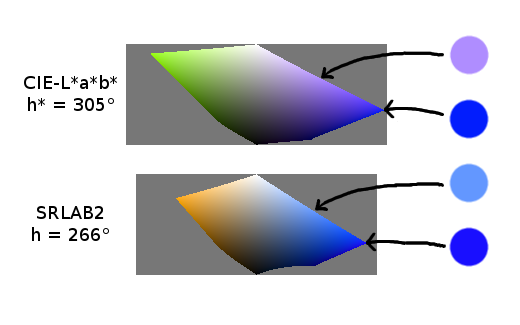# SRLAB2an alternative to CIE-L*a*b*The CIE-L*a*b* model in polar coordinates is a widespread standard to represent colors in terms of lightness (L*), chroma (c*) and hue (h*). All colors with the same L* value but different c* and h* values should appear same bright; all colors with the same c* value but different L* and h* values should appear same colorful; all colors with the same h* value but different L* and c* values should have the same color-hue. In practice the CIELAB model does NOT fulfill these critera. An example is given on the right.

There are more advanced color models like CIECAM02, but these models are far more complicated to implement and to use, as they depend on ambient lighting, absolute luminance, etc. “SRLAB2” uses transformations of CIECAM02 to fix some of the deficiencies of CIELAB while maintaining almost CIELAB's simplicity.

Mathematical details about the problems of CIELAB and the definition of the new model SRLAB2 can be found in my PDF-Paper (LaTeX source).

There are also some videos showing the sRGB gamut in the SRLAB2 color space:

The following C-code can be used to transform sRGB values (represented as double precision floats between 0.0 and 1.0) to SRLAB2 values (rectangular) and vice versa. Note that the code below maps sRGB #000000 to L* = 0, disregarding sRGB's encoding viewing flare of 1%.

```void rgb_to_lab(
double red, double green, double blue,
double *lightness, double *a, double *b
) {
double x, y, z;
if (red <= 0.03928) red /= 12.92;
else red = pow((red + 0.055) / 1.055, 2.4);
if (green <= 0.03928) green /= 12.92;
else green = pow((green + 0.055) / 1.055, 2.4);
if (blue <= 0.03928) blue /= 12.92;
else blue = pow((blue + 0.055) / 1.055, 2.4);
x = 0.320530 * red + 0.636920 * green + 0.042560 * blue;
y = 0.161987 * red + 0.756636 * green + 0.081376 * blue;
z = 0.017228 * red + 0.108660 * green + 0.874112 * blue;
if (x <= 216.0/24389.0) x *= 24389.0 / 2700.0;
else x = 1.16 * pow(x, 1.0/3.0) - 0.16;
if (y <= 216.0/24389.0) y *= 24389.0 / 2700.0;
else y = 1.16 * pow(y, 1.0/3.0) - 0.16;
if (z <= 216.0/24389.0) z *= 24389.0 / 2700.0;
else z = 1.16 * pow(z, 1.0/3.0) - 0.16;
*lightness =    37.0950 * x +  62.9054 * y -   0.0008 * z;
*a         =   663.4684 * x - 750.5078 * y +  87.0328 * z;
*b         =    63.9569 * x + 108.4576 * y - 172.4152 * z;
}

void lab_to_rgb(
double lightness, double a, double b,
double *red, double *green, double *blue
) {
double x, y, z, rd, gn, bl;
x = 0.01 * lightness + 0.000904127 * a + 0.000456344 * b;
y = 0.01 * lightness - 0.000533159 * a - 0.000269178 * b;
z = 0.01 * lightness                   - 0.005800000 * b;
if (x <= 0.08) x *= 2700.0 / 24389.0;
else x = pow((x + 0.16) / 1.16, 3.0);
if (y <= 0.08) y *= 2700.0 / 24389.0;
else y = pow((y + 0.16) / 1.16, 3.0);
if (z <= 0.08) z *= 2700.0 / 24389.0;
else z = pow((z + 0.16) / 1.16, 3.0);
rd =  5.435679 * x - 4.599131 * y + 0.163593 * z;
gn = -1.168090 * x + 2.327977 * y - 0.159798 * z;
bl =  0.037840 * x - 0.198564 * y + 1.160644 * z;
if (rd <= 0.00304) *red = rd * 12.92;
else *red = 1.055 * pow(rd, 1.0/2.4) - 0.055;
if (gn <= 0.00304) *green = gn * 12.92;
else *green = 1.055 * pow(gn, 1.0/2.4) - 0.055;
if (bl <= 0.00304) *blue = bl * 12.92;
else *blue = 1.055 * pow(bl, 1.0/2.4) - 0.055;
}```

You can freely use, copy, modify, merge, publish, distribute, etc. these code snippets. There is no warranty of any kind: Use at your own risk.